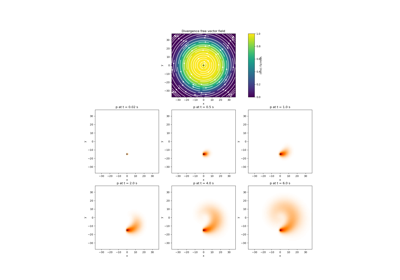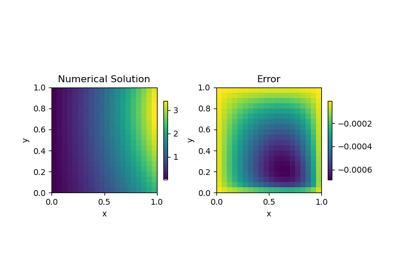# discretize.base.BaseTensorMesh#class discretize.base.BaseTensorMesh(h, origin=None, **kwargs)[source]#

Base class for tensor-product style meshes.

This class contains properites and methods that are common to Cartesian and cylindrical meshes. That is, meshes whose cell centers, nodes, faces and edges can be constructed with tensor-products of vectors.

Do not use this class directly! Practical tensor meshes supported in discretize will inherit this class; i.e. discretize.TensorMesh and CylindricalMesh. Inherit this class if you plan to develop a new tensor-style mesh class (e.g. a spherical mesh).

Parameters:
h(dim) iterable of int, numpy.ndarray, or tuple

Defines the cell widths along each axis. The length of the iterable object is equal to the dimension of the mesh (1, 2 or 3). For a 3D mesh, the list would have the form [hx, hy, hz] .

Along each axis, the user has 3 choices for defining the cells widths:

• int -> A unit interval is equally discretized into N cells.

• numpy.ndarray -> The widths are explicity given for each cell

• the widths are defined as a list of tuple of the form (dh, nc, [npad]) where dh is the cell width, nc is the number of cells, and npad (optional) is a padding factor denoting exponential increase/decrease in the cell width for each cell; e.g. [(2., 10, -1.3), (2., 50), (2., 10, 1.3)]

origin(dim) iterable, default: 0

Define the origin or ‘anchor point’ of the mesh; i.e. the bottom-left-frontmost corner. By default, the mesh is anchored such that its origin is at [0, 0, 0].

For each dimension (x, y or z), The user may set the origin 2 ways:

• a scalar which explicitly defines origin along that dimension.

• {‘0’, ‘C’, ‘N’} a str specifying whether the zero coordinate along each axis is the first node location (‘0’), in the center (‘C’) or the last node location (‘N’).

utils.unpack_widths

The function used to expand a list or tuple to generate widths.

Attributes

 boundary_edges Boundary edge locations. boundary_face_outward_normals Outward normal vectors of boundary faces. boundary_faces Boundary face locations. boundary_nodes Boundary node locations. cell_centers Return gridded cell center locations. cell_centers_x Return x-coordinates of the cell centers along the x-direction. cell_centers_y Return y-coordinates of the cell centers along the y-direction. cell_centers_z Return z-coordinates of the cell centers along the z-direction. edges Gridded edge locations. edges_x Gridded x-edge locations. edges_y Gridded y-edge locations. edges_z Gridded z-edge locations. faces Gridded face locations. faces_x Gridded x-face locations. faces_y Gridded y-face locations. faces_z Gridded z-face locations. h Cell widths along each axis direction. h_gridded Return dimensions of all mesh cells as staggered grid. hx Width of cells in the x direction. hy Width of cells in the y direction. hz Width of cells in the z direction. nodes Return gridded node locations. nodes_x Return x-coordinates of the nodes along the x-direction. nodes_y Return y-coordinates of the nodes along the y-direction. nodes_z Return z-coordinates of the nodes along the z-direction. origin Origin or 'anchor point' of the mesh. vectorCCx vectorCCx has been deprecated. vectorCCy vectorCCy has been deprecated. vectorCCz vectorCCz has been deprecated. vectorNx vectorNx has been deprecated. vectorNy vectorNy has been deprecated. vectorNz vectorNz has been deprecated.

Methods

 getTensor(*args, **kwargs) getTensor has been removed. get_interpolation_matrix(loc[, ...]) Construct a linear interpolation matrix from mesh. get_tensor(key) Return the base 1D arrays for a specified mesh tensor. isInside(*args, **kwargs) isInside has been removed. is_inside(pts[, location_type]) Determine which points lie within the mesh.

## Galleries and Tutorials using discretize.base.BaseTensorMesh#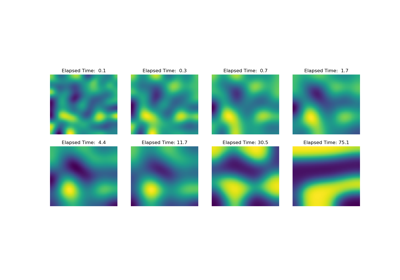Operators: Cahn Hilliard

Operators: Cahn Hilliard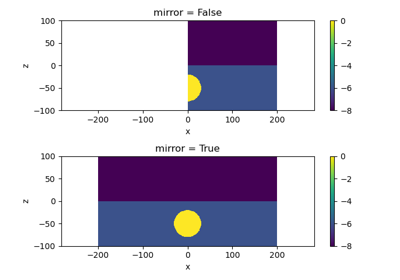Plot Mirrored Cylindrically Symmetric Model

Plot Mirrored Cylindrically Symmetric Model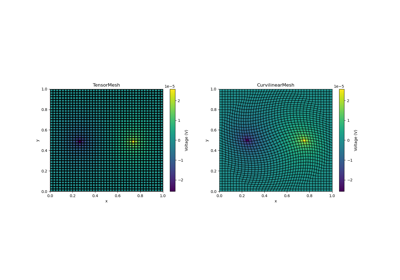Basic Forward 2D DC Resistivity

Basic Forward 2D DC ResistivityBasic: PlotImage

Basic: PlotImage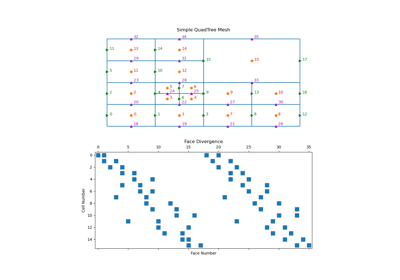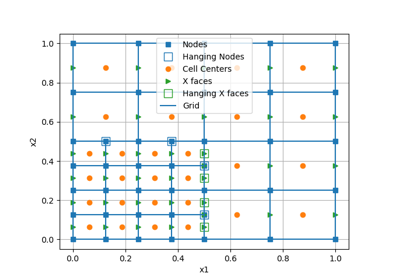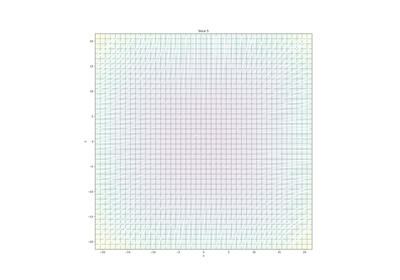Plotting: Streamline thickness

Plotting: Streamline thickness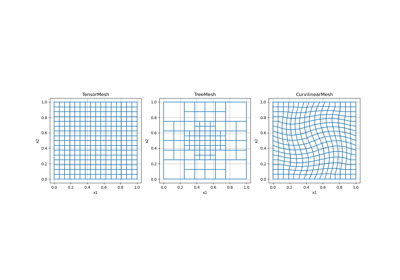Overview of Mesh Types

Overview of Mesh Types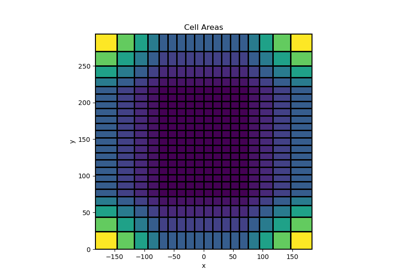Tensor meshes

Tensor meshes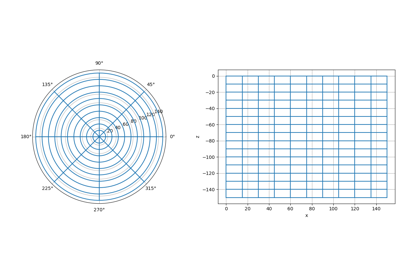Cylindrical meshes

Cylindrical meshes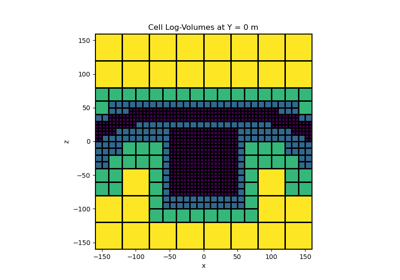Tree Meshes

Tree Meshes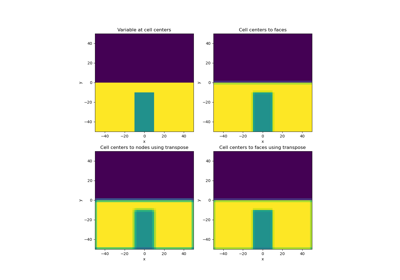Averaging Matricies

Averaging Matricies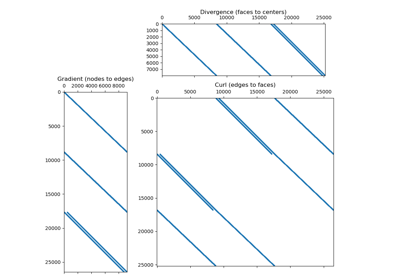Differential Operators

Differential Operators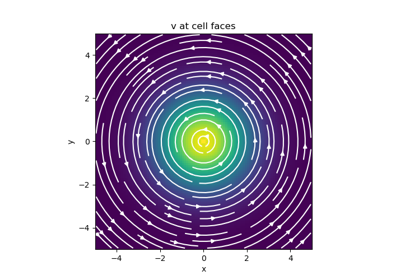Basic Inner Products

Basic Inner Products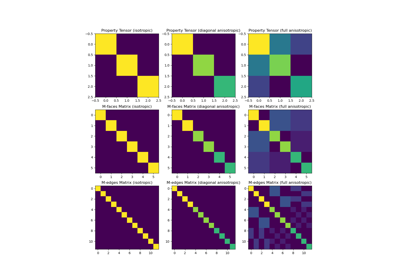Constitutive Relations

Constitutive Relations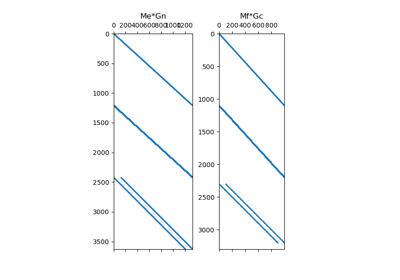Differential Operators

Differential Operators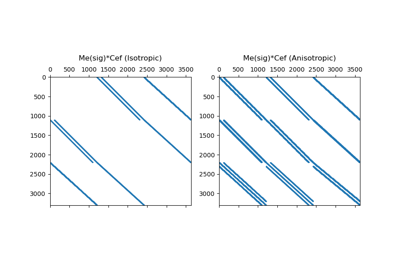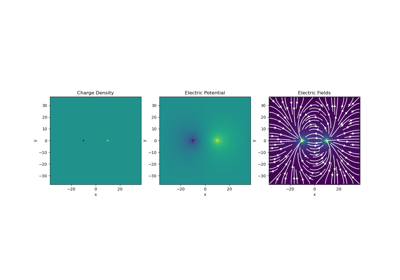Gauss’ Law of Electrostatics

Gauss' Law of Electrostatics